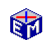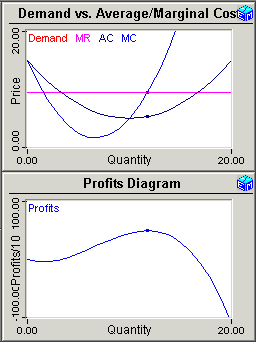Perfect Competition

The Perfect Competition application first draws and explains the origins of (a) the average cost curve, (b) the marginal cost curve, (c) the demand curve, and (d) the marginal revenue curve.Model Link:  Perfect Competition
<activate the model links>
Printable PDF ExercisesQ: When is MC is above AC, below AC, and equal to AC?

The next step is to find the profit-maximizing position.  You adjust the quantity produced manually to see first-hand how a firm facing a horizontal demand curve maximizes profits by producing where the marginal cost equals the market price.  You then use a built-in iterative procedure to automatically maximize profits.

Movie:  Perfect Competition
(40 seconds)

To illustrate the behavior of the firm in a changing environment, you study how the profit maximizing quantity changes when changes in technology shift the average cost and marginal cost curves.

Classic Economic Models

Microeconomics

Introduction
Overview of Micro Models

Macroeconomics

Introduction
Overview of Macro Models

Resources